Section A

# A Flow Analysis of Small Craft by Using CFD

Ji-Yong Park1, Jin-Hee Jeong1, Tea-Wook Hwang1, Sol-Ah Lee1, Kyung-Sung Kim1,*
1School of Naval Architecture and Ocean Engineering, Tongmyong University, Busan, Korea, {jiyong@tu.ac.kr, jinhee@tu.ac.kr, TWhang@tu.ac.kr, sola@tu.ac.kr}
*Corresponding Author: Kyung Sung Kim, 428 Sinseon-Ro, Nam-Gu, Busan, Korea, +82-51-629-1655, keiuskim@tu.ac.kr.

© Copyright 2020 Korea Multimedia Society. This is an Open-Access article distributed under the terms of the Creative Commons Attribution Non-Commercial License (http://creativecommons.org/licenses/by-nc/4.0/) which permits unrestricted non-commercial use, distribution, and reproduction in any medium, provided the original work is properly cited.

Received: Dec 05, 2020; Revised: Dec 23, 2020; Accepted: Dec 27, 2020

Published Online: Dec 31, 2020

## Abstract

The small craft including jet-board for leisure are commonly smaller than the general commercial vessels. For the floating vessel, the motion analysis is significantly important component to design the shape. It is, however, hardly predicting its behavior by using conventional boundary element method due to violating small amplitude assumption for potential theory. The computational fluid dynamics method can afford to simulate such small craft, but its grid system was not able to calculate motion, because movable body disturbs the grid system by confliction. The dynamics fluid body interaction model with over-set mesh system can be dealt with movable floating body under irregular ocean wave. In this study, several cases were considered to reveal that DFBI is essential method to predict floating body motion. The single phase simulate was conducted to establish the shape perfection, and then the validated vessel was simulated with ocean waves weather DFBI option on or off. Through the comparison, the results between the cases of DFBI on and off shows significantly difference. It was claimed that the DFBI was necessary not only to calculation body motion, but also to predict accurate drag and lift force on the floating body for small size craft.

Keywords: Computational fluid dynamics; Dynamic Body Fluid Interaction; Motion under waves; Small craft motion

## I. INTRODUCTION

For many decades ago, the leisure activities on the ocean and river have been dramatically changed due to social demand. The ocean leisure, especially, extended its territory from group activities to individual activities with new technology including electronics power vessel . These vessels have smaller hull size compare to the commercial logistic ship such as crude cargo ship or LNG (Liquified Natural Gas) carrier.

The general size vessel such as LNG carrier can be simulated by BEM (Boundary Element Method) based on potential theory. In the BEM simulation, the vessel motion can be predicted in both of frequency and time domain. For the frequency domain, the motion at each frequency were obtained by using panel method . The time domain simulation used Volterra integral method considering time memory effect . These frequency and time domain simulation were developed with respect to the potential theory which has assumption of irrotational, inviscid and incompressibility .

The virtue of potential theory is fast calculation, however, its characteristic such as small amplitude assumption may be useless for small size craft . In this regard, the computational fluid dynamics (CFD) method was employed to simulate small craft behavior under relatively short-wave period. The CFD can estimate force and moments on the floating body properly . However, the grid system may be disturbed due to vessel movement. Therefore, the floating body effects which is radiation and diffraction effects cannot be considered . The only stationary vessel was located at the initial position; thus, it cannot be predicting floating body effect accurately.

Recently, Dynamic Fluid Body Interaction (DFBI) method was introduced including over-set mesh system. The DFBI enables the analysis of vessel when the motion is not known priori [7,8]. When the fluid and external forces act on the vessel, the motion of vessel can be imparted through the over-ser mesh. As results, it is possible to simulate movable object situation without computational error. Moreover, the DFBI contact coupling model allows bodies to collide and rebound from contact which is modeled as a repulsive force [9,10].

In this study, the small craft such as leisure jet board was analyzed with STARCCM+ 15.06. For the analysis, the single-phase problem for shape feasibility, multi-phase problem for resistance and DFBI problem for accurate resistance calculation under real ocean situation were conducted.

## II. NUMERICAL MRTHODOLOGY

In this study, STAR-CCM+ 15.06 which is one of most well-known commercial CFD program conducted to simulate flow under regular waves. For the CFD, the continuity and the Navier=Stokes’ equations were employed as governing equations as follows:

$\frac{D\rho }{Dt}=0$
(1)
$\frac{Du}{Dt}=-\frac{1}{\rho }\nabla P+v{\nabla }^{2}u+F$
(2)

where ρ is density, t is time, u is velocity, ∇ is gradient, v is dynamics viscosity coefficient, ∇2 is Laplacian and F is external forces.

When object is located on the free-surface, the free-surface layer can be disturbed due to collision between object and fluid, and then the large deformation of free-surface can be observed. In this case, the flow turns into the turbulence region, thus turbulence model was employed. Among the many models of turbulence, the SST k–ω was chosen. The SST k–ω model is hybrid model which combined k–ω and k–ε model. It has advantage to expect the flow far away from the object and it can be expressed as follows:

$\frac{D\omega }{Dt}=\nabla \cdot \left(\frac{{v}_{T}}{{\sigma }_{\omega }}\nabla \omega \right)+\left({C}_{\omega 1}-1\right)\frac{{P}_{\omega }}{k}-\left({C}_{\omega 2}-1\right){\omega }^{2}+\frac{2{v}_{T}}{{\sigma }_{\omega }k}\nabla \omega \cdot \nabla k$
(3)

where ω is energy dissipation rate, vT is turbulence dynamic viscosity coefficient, σ is Prandtl number of turbulence dissipation rate, C is arbitrary constant and k is turbulence kinetic energy. Because of using turbulence model, the Governing equations can be re-written as follows:

$\frac{\partial \rho }{\partial t}+\frac{\partial }{\partial x}\left(\rho u\right)=0$
(4)
$\frac{\partial \rho u}{\partial t}+\frac{\partial }{\partial {x}_{j}}\left(\rho {u}_{i}{u}_{j}\right)=-\frac{\partial P}{\partial {x}_{i}}+\frac{\partial {\tau }_{ij}}{\partial {x}_{j}}$
(5)
$\frac{\partial \rho E}{\partial t}+\frac{\partial }{\partial {x}_{j}}\left(\rho H{u}_{j}\right)=\frac{\partial }{\partial {x}_{j}}\left(k\frac{\partial T}{\partial {x}_{j}}\right)+\frac{\partial \left[u\cdot {\tau }_{ij}\right]}{\partial {x}_{j}}$
(6)

where x is axis of coordination, τ is shear stress, E is energy, T is time scale, H is kinetic energy dissipation rate. Eq. (5). denotes momentum equation for turbulence and Eq. (6). denotes energy equation for turbulence. Both the momentum and energy equations were derived from Navier-Stokes’ equation. The shear stress can be calculated as follows:

${\tau }_{ij}=\mu \left(2{S}_{ij}-\frac{2}{3}{S}_{kk}{\delta }_{ij}\right)$
(7)

where

${S}_{ij}=\frac{1}{2}\left(\frac{\partial {u}_{i}}{\partial {x}_{j}}+\frac{\partial {u}_{j}}{\partial {x}_{i}}\right)$
(8)

## III. SIMULATION RESULTS

In this study, jet board which is small leisure craft with propulsion system shown in Fig. 1. Fig. 2. represents its schematic model for numerical simulation. The characteristics of jet board is given in Table 1. For the numerical computational domain shown in Fig. 3 has 18L length, 6W width and 20D height where L is length W is width and D is depth of jet board.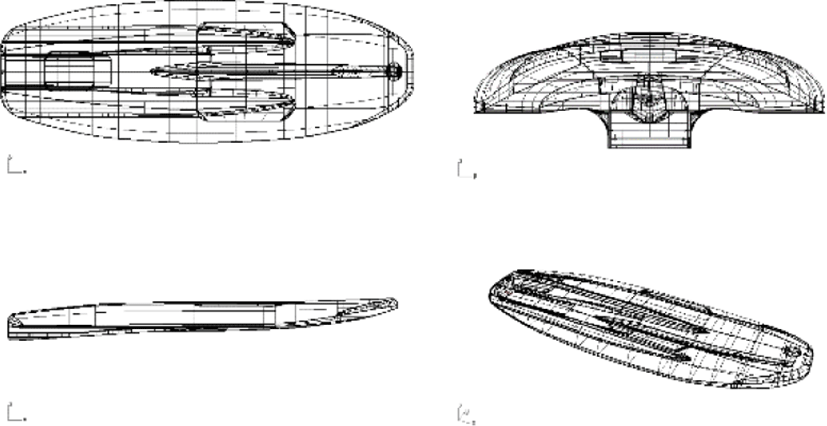Fig. 1. CAD model for jet board.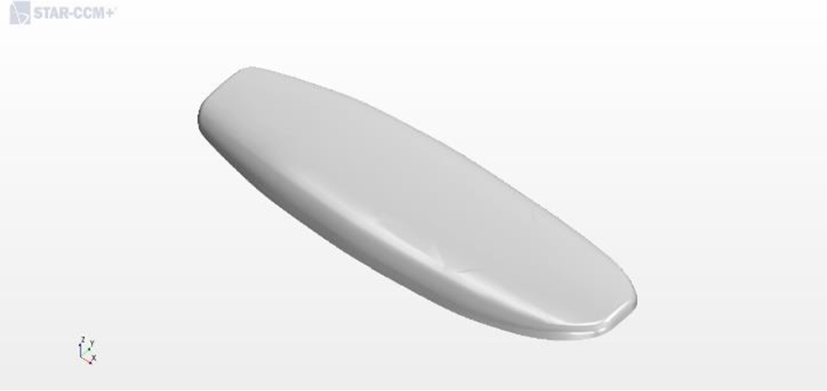Fig. 2. Schematic model for numerical simulation.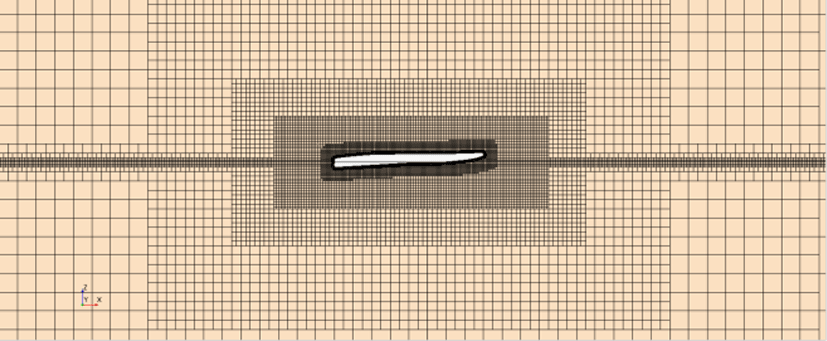Fig. 3. Example of Computational domain with grid.
Table 1. Characteristics of Jet Board.
Characteristics Magnitude
Length [m] 2.1
Height [m] 0.2
Width [m] 0.65
Draft [m] 0.1
Volume [m3] 0.1178
Mass [kg] 70.6782
Block Coefficient 0.287
Center of Gravity x-dir 1.00717
y-dir 0
z-dir 0.04946
Moment of Inertia Ixx 0.002943
Iyy 0.032478
Izz 0.035099
Mesh for Calculation x+ 8L
x− 10L
y+ 3W
y− 3W
z+ 10D
z− 10D

For the grid modelling for simulation, Trimmer cell Mesher and prism layer Mesher provided by STARCCM+ were selected. For the calculation approximately 900,000 cells were used and flexible time interval by CFL condition were employed.

In this investigation, there is two stage: firstly, using single phase flow to analyze shape evaluation, and secondly to estimate proper resistance force under regular incidence waves. The specific simulation conditions can be found in Table 2.

Table 2. Simulation conditions for each case.
Case Body Position Wave Profile Flow Phase Body Dynamics
1 Fixed None Single None
2 Fixed Numerical Flatwave Multi None
3 Fixed Numerical First order Wave Multi None
4 Free Floating Numerical First order Wave Multi DFBI

Fig. 4 represents the pressure distribution on the bottom of jet board. Fig. 4 (a)~(d) shows the pressure contour at time 1.0 sec and Fig. 4 (e)~(h) shows the pressure contour at time 10.0 sec. As shown in the Fig. 4, the pressure distribution of case 1 shows that the shape feasibility was obtained. No sudden change of pressure on the body was observed. However, in Case 2-4, the simulation was performed under the condition of real ocean environment including free surface, a concentrated load was observed on the bottom of the vessel. Since the front of the vessel was not attached to the fluid, there is no pressure, however, the concentrated pressure was observed the bottom of vessel due to contacting with free surface.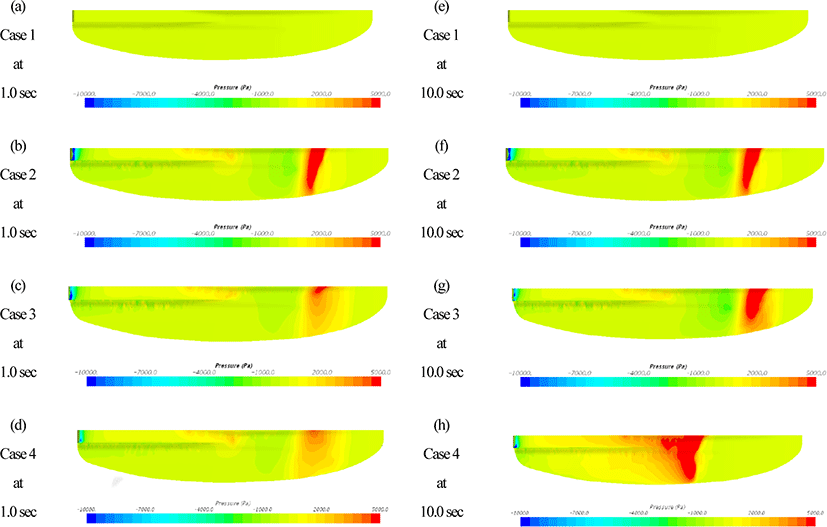Fig. 4. Pressure contour on floating body at various time for each case.

Comparing Case 2-4, where pressure is generated by contact, they show almost the same aspect in the early stages of the simulation. The difference is that in Case 2, which does not physically create ocean waves, isobars are formed along the free-surface, but in Case 3, make sure that the pressure is concentrated on the keel protrusions. In Case 4, it can be confirmed that the pressure is concentrated in the keel part, and it can be confirmed that the distribution is slightly different from that in Case 3, but the difference was not large in the initial simulation. As can be seen from the comparison in Fig. 4, it was not possible to see the change in the pressure distribution with the time progress compare to Case 2 and 3. In the case of Case 4, it was shown that the position of the concentrated pressure changed in the pressure distribution. Using DFBI, the movement of the fluid distributions were predicted according to the shape and pressure of the wave. It comes to have a substantial movement, and it is caused by the difference in the point where the fluid meets the vessel body. In fact, there is no difference when ocean waves that are relatively small compared to the size of the ship are encountered, but in the case of small ships such as jet boards, changes were considered significantly.

Fig. 5 shows that the velocities of the fluid measured at 5 seconds and 10 seconds from each case. As can be inferred from the pressure comparison, it shows that the laminar flow is formed in Case 1 without a large fluctuation due to the no sudden changes in velocity field which mean there is no disturbance of body motion due to flows. It can be said that the shape of the fluid is well designed. However, in Cases 2 to 4, cases of free surface exists, the phenomenon of air stagnation between the free surface and the partial fluid can be confirmed, and vortices are formed in vessel heading due to these congestions. These vortices mean that the hull design is improper when interpreted solely in Case 2 and Case 3 as being capable of inducing vibrations in the body.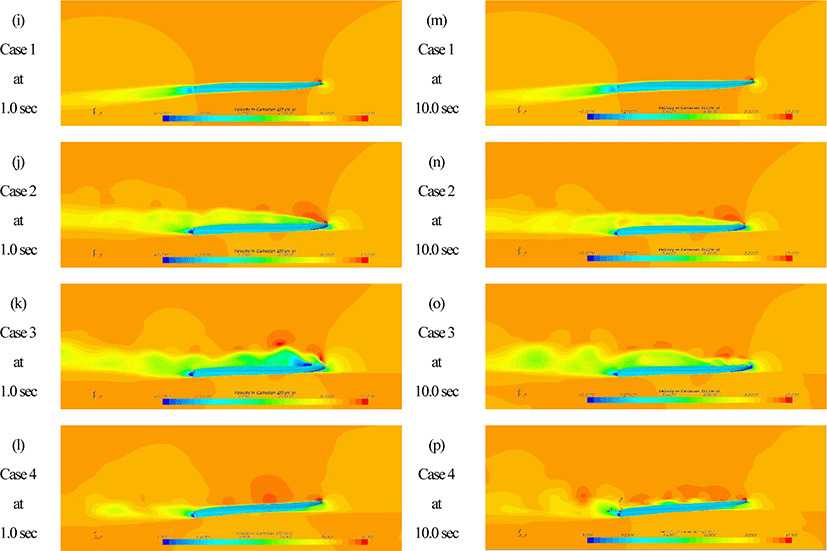Fig. 5. Velocity profile near floating body at various time for each case.

However, in the case of Case 4, which considers the actual movement of the ship, the effect of the trap between the free surface and the body was suppressed by the movement of the ship. The velocity field and streamline were close to that of the Case 1. This is because the change in movement may not be dominant without considering the movement of the ship when reproducing the ocean waves using VOF or first order used in general large ships. There was no problem with large vessel, but it was dominant component to the small craft such as a jet board which does not follow the small amplitude assumption, so it has a great effect on the movement of the hull. According to the Case 2 and Case 3, the shape of vessel was need to be modified considering the suitability of the hull design. However, the result of case 4 which is the simulation under real waves with movable floating body system, there is no point to be modified its shape. It means if researcher follows conventional method, the entire design can be wrong with wrong simulation results, especially small craft.

Table 3 shows the drag and lift in each case. As can be seen from the table, the drag and lift are low when the fluid is assumed to be air even though it is a single-phase flow. In the case of a simulation that considers the free surface that actually operates in a floating body, reproduce the numerical VOF wave instead of the actual wave, and confirm that it came out lower than Cases 3 to 4. This is a study of the force that the slamming effect that occurs when an actual wave meets a negative fluid is not applied, and the floating body is repelled by the reaction force due to collision with the free surface. A comparison of velocity field shows that Case 4 was more stable than Case 3, however, that Case 4 was about 4.5 times higher in terms of practical drag and lift. It is shown that this is difference caused by the change in the angle of impacting the wave while moving abundantly and the generation of room dead force due to the wave, and by this, in the case of small fluid, a method using DFBI in interpreting drag and lift.

Table 3. Table of Forces on the floating body for each case.
Index Single Phase VOF Flat Wave First Order Wave First order Wave with DFBI
Lift [N] Pressure min. 2.01249 155.1495 136.062 398.8764
max. 2.012553 168.9885 237.0171 1127.674
Ave. 2.012522 160.5529 186.1345 692.7336
Shear min. -0.00861 -0.39236 -0.47405 -1.92888
max. -0.00861 -0.35857 -0.2486 -0.87481
Ave. -0.00861 -0.37461 0.33875 -1.53559
Total min. 2.003878 154.772 135.6672 397.5321
max. 2.00394 168.6187 236.6585 1126.325
Ave. 2.003909 160.1783 185.7957 691.198
Drag [N] Pressure min. 0.234696 20.15397 13.52569 25.15113
max. 0.234742 20.99275 31.33211 61.26003
Ave. 0.234721 20.59444 20.81277 44.81347
Shear min. 0.435368 17.64843 13.0609 14.84456
max. 0.435368 18.41477 21.68638 28.41618
Ave. 0.435368 18.02457 16.63149 21.41678
Total min. 0.670064 37.92858 27.01605 41.66773
max. 0.67011 39.27033 51.35952 89.48196
Ave. 0.670089 38.61901 37.44426 66.23025

## IV. CONCLUDING REMARKS

In this study, we predicted the drag and lift of small fluids at sea using a grid-based computational fluid dynamics method. The STAR-CCM + program was used for this study, and studies were conducted under various simulation conditions. When set up for this study, 1) single-phase flow, 2) multi-phase flow (Case of VOF wave and fixed body), 3) multi-phase flow (Case of First order wave and fixed body) 4) multi-phase flow (Case of First order wave and DFBI) was used.

In general, Case 2 or Case 3 conducted to estimate ship drag and lift force. However, in the case of small fluids, it is not possible to establish the small amplitude assumption, so it is important to consider it for the movement of the ship. As a result, the simulation results in each case were compared with the difference due to the pressure distribution and the difference in the velocity field. As a result, the same result that the hull design when the movement of the ship was not taken into consideration was inappropriate was shown. However, in the case of a simulation that considers the movement of the ship using DFBI, it was shown that the flow around the hull appears in the same way as the result of the wind tunnel experiment using single-phase flow.

When comparing the overall cases, in this study, 1) single flow, 2) multi-phase flow VOF Flat wave 3) multi-phase flow f First order wave is assumed and moves at 4 m / s. A flow simulation was performed on the award-winning movement chain jet board.

In the case 1, it is possible to analyze the flow caused by the shape of the object and investigate the overall design. As a conclusion in case 1, in the case of this jet board, the characteristics of a flat board in the general Slender form were clearly shown. it shows that there was no part that caused singular flow with the appearance of an object.

In the case 2, the drag and lift under wave were estimated using the VOF Flat wave. It showed very high drag and lift values compared to a single flow, but it was induced by the free surface effect of this and the increase in pressure due to the crushing that occurs between the free surface and the object.

In the case 3, we used the First order wave to implement waves those were closer to reality than the VOF wave. This confirmed that it was possible to simulate the slamming phenomenon that occurs when the waves were investigated, and then, the pressure increasing was observed. However, in the First order wave, the vortex of air generated in vessel head which promotes turbulence flow. and shows a phenomenon that causes initial separation, and then it may reduce drag force. It was. However, the increased flow due to crushing caused contact surfaces and wakes, the overall drag was higher in the case of the first order wave, and the lift was also higher due to the slamming and free surface effect.

In case 4, DFBI was used for the first order wave to reproduce and simulate more realistically than the previous case 2 and case 3. Both drag and lift force were increases due to movable body and its motion, however, the streamline was more stable than the case 2 and case 3. It shows that case 2 and case 3 claim that the hull design was need to be modified, but the case 4 verified the hull design was perfection as shown in the case 1.

Through the comparison among each case, it was shown that the drag and lift force had significant difference even the same vessel was used. It was revealed that the small craft such as leisure boat or Jet-board DFBI method provided more accurate results. In the future work, the developed DFBI system will be simulate small craft behavior with smart vessel device such as automatic maneuvering and enhanced see-keeping equipment with IoT (Internet of Things) device. It would be very useful to validate the smart device on the vessel to predict its effectiveness numerically.

## REFERENCES

.

Kim, M.H., “Difference-frequency wave loads on a large body in multidirectional waves,” Applied Ocean Research, vol. 14, pp. 353-370, 1992..

Kim, K.S. and Kim, M. H., “Dynamic coupling between ship motion and three-layer-liquid separator by using moving particle simulation,” International journal of offshore and polar engineering, vol. 24, no. 2 pp. 122-128, 2014..

ITTC, “Report of performance committee,” the 15th International Towing Tank Committee, Hague, 1977..

Kim, I. S., Park, D. W. and Lee, S. B., “Prediction of fishing boat performance using computational fluid dynamics,” Journal of the Korea Society of Marine Engineering, vol. 40, no. 7, pp. 574-579, 2016..

Park, D. W. and Kim, K. S., “Assessment of the energy efficiency performance by the fore-body retrofit of the coastline,” Journal of Korean Society of Marine Environment and Safety, vol. 23, no. 7. pp. 965-971., 2017..

Yang, H. Y., Kim, B. H., Yoo, J.H. and Kim, W.J., “Wake comparison between model and full scale ships using CFD,” Journal of the Society of Naval Architects of Korea, vol. 47, no. 2, pp. 150-162, 2010..

Park, D.W., Lee, S.B., Chung, S.S., Seo, H.W. and Kwon, J.W., “Effects of trim on resistance performance of a ship,” Journal of the Society of Naval Architects of Korea, vol. 50, no. 2, pp. 88-94, 2013..

Zhang, Y. and Kim, B. “A Fully coupled computational fluid dynamics method for analysis of semi-submersible floating offshore wind turbines under wind-wave excitation conditions based on OC5 data,” Applied Sciences, vol. 8, no. 11, 2018..

Kim, G.H. and Park, S. “Development of a numerical simulation tool for efficient and robust prediction of ship resistance,” International Journal of Naval Architecture and Ocean Engineering, vol 9, no. 5, pp. 537-551, 2017.## Authors

Kyung Sung Kimis Assistant Professor of Naval Architecture and Ocean Engineering at Tongmyong University, Busan, Korea. He received bachelors and master degrees in n Naval Architecture and Ocean Engineering from Pusan National University In Korea. He then moved to Texas, U.S.A. and earned a Ph.D. in Ocean engineering from the Texas A&M University. He was employed at the Pohang University of Science and Technology as research professor. His major field is the hydrodynamics and computational fluid dynamics including particle method. However, he now is in charge of experimental approaches to offshore experiments for education. He is working to develop efficient methods of educating young researchers with fourth industrial revolution technology in both numerical and experimental research.

Ji-Yong Parkis senior bachelor student in School of Naval Architecture and Ocean Engineering at Tongmyong University. His research area is Computational Fluid Dynamics and Modelling.

Jin-Hee Jeongis senior bachelor student in School of Naval Architecture and Ocean Engineering at Tongmyong University. His research area is Theoretical Fluid Dynamics and Mathematical Modelling.

Tae-Wook Hwangis a junior bachelor student in School of Naval Architecture and Ocean Engineering at Tongmyong University. His research area is Computational Fluid Dynamics and Modelling.

Sol-Ah Leeis a junior bachelor student in School of Naval Architecture and Ocean Engineering at Tongmyong University. His research area is Computational Fluid Dynamics and Modelling.